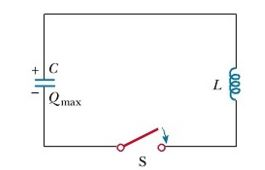# LC Oscillations

An LC circuit, also called a resonant circuit, tank circuit, or tuned circuit, is an electric circuit consisting of an inductor, represented by the letter L, and a capacitor, represented by the letter C, connected together. The circuit can act as an electricalresonator, an electrical analogue of a tuning fork, storing energy oscillating at the circuit's resonant frequency.

A circuit that has only a capacitor and an inductor. We shall pretend that these components are ideal, ie that they have no internal resistance. In practice this is not possible: there will always be some way in which electrical energy can be converted to heat, even if the rate is very small. We begin by charging the capacitor C with an initial charge qo. (In the demonstration shown in the photograph above, we did this by connecting the capacitor to a battery, then disconnecting it.) We then connect the capacitor to an inductance L, as shown below, and analyse the current i that flows and the voltage V across the components.The oscillations of the LC circuit are an electromagnetic analog to the mechanical oscillations of a block– spring system, which we studied earlier. Much of what we discussed is applicable to LC oscillations. For example, we investigated the effect of driving a mechanical oscillator with an external force, which leads to the phenomenon of resonance. We observe the same phenomenon in the LC circuit. For example, a radio tuner has an LC circuit with a natural frequency, which we determine as follows: When the circuit is driven by the electromagnetic oscillations of a radio signal detected by the antenna, the tuner circuit responds with a large amplitude of electrical oscillation only for the station frequency that matches the natural frequency. Thus, only the signal from one station is passed on to the amplifier, even though signals from all stations are driving the circuit at the same time. When you turn the knob on the radio tuner to change the station, you are changing the natural frequency of the circuit so that it will exhibit a resonance response to a different driving frequency.